﻿ 利用示教学习的移动机械臂轨迹避障算法
«上一篇文章快速检索 高级检索

 哈尔滨工程大学学报2018, Vol. 39Issue (9): 1546-1553  DOI: 10.11990/jheu.2017030300

### 引用本文LIU Weihui, CHEN Diansheng, ZHANG Lizhi. Learning from demonstration based obstacle avoidance algorithm to plan the trajectory of a mobile manipulator[J]. Journal of Harbin Engineering University, 2018, 39(9), 1546-1553. DOI: 10.11990/jheu.201703030.### 文章历史

Learning from demonstration based obstacle avoidance algorithm to plan the trajectory of a mobile manipulator
LIU Weihui, CHEN Diansheng, ZHANG Lizhi
School of Mechanical Engineering and Automation, Beihang University, Beijing 100191, China
Abstract: To improve the environmental adaptability of service robots and alleviate user loads, a trajectory amendment algorithm utilizing learning from demonstration is proposed in this paper. First, a new trajectory with a shape similar to that previously demonstrated was generated by utilizing the dynamic movement primitives model, after which an improved distance-weighted k-nearest neighbor algorithm was developed to realize local modification for the trajectory shape at the end of the mobile manipulator. An online updating method was designed to avoid loss of adjacent effective training data. Obstacle avoidance experiments and real-time tests were then implemented in the human-robot interaction system. The experimental results showed the adaptability of the proposed obstacle avoidance algorithm to the new task, the obstacle avoidance decision ability and the online modification ability, to ensure friendly and smooth human-robot interactions.
Keywords: service robot    learning from demonstration    human-robot interaction    mobile manipulator    obstacle avoidance for trajectory    online adjusting    k-nearest neighbor algorithm    dynamic movement primitives

1 基于DMP模型的轨迹生成

DMP[12-13]通过加有外部干扰项的线性弹簧阻尼系统实现对任意形状轨迹的动作表达。每个独立自由度上的动力学表达式如下

 $\tau \dot v = K\left( {g - x} \right) - Dv + f\left( s \right)$ (1)
 $\tau \dot x = v$ (2)

 $f\left( s \right) = \frac{{\mathop \sum \limits_i {\omega _i}{\psi _i}\left( s \right)s}}{{\mathop \sum \limits_i {\psi _i}\left( s \right)}}$ (3)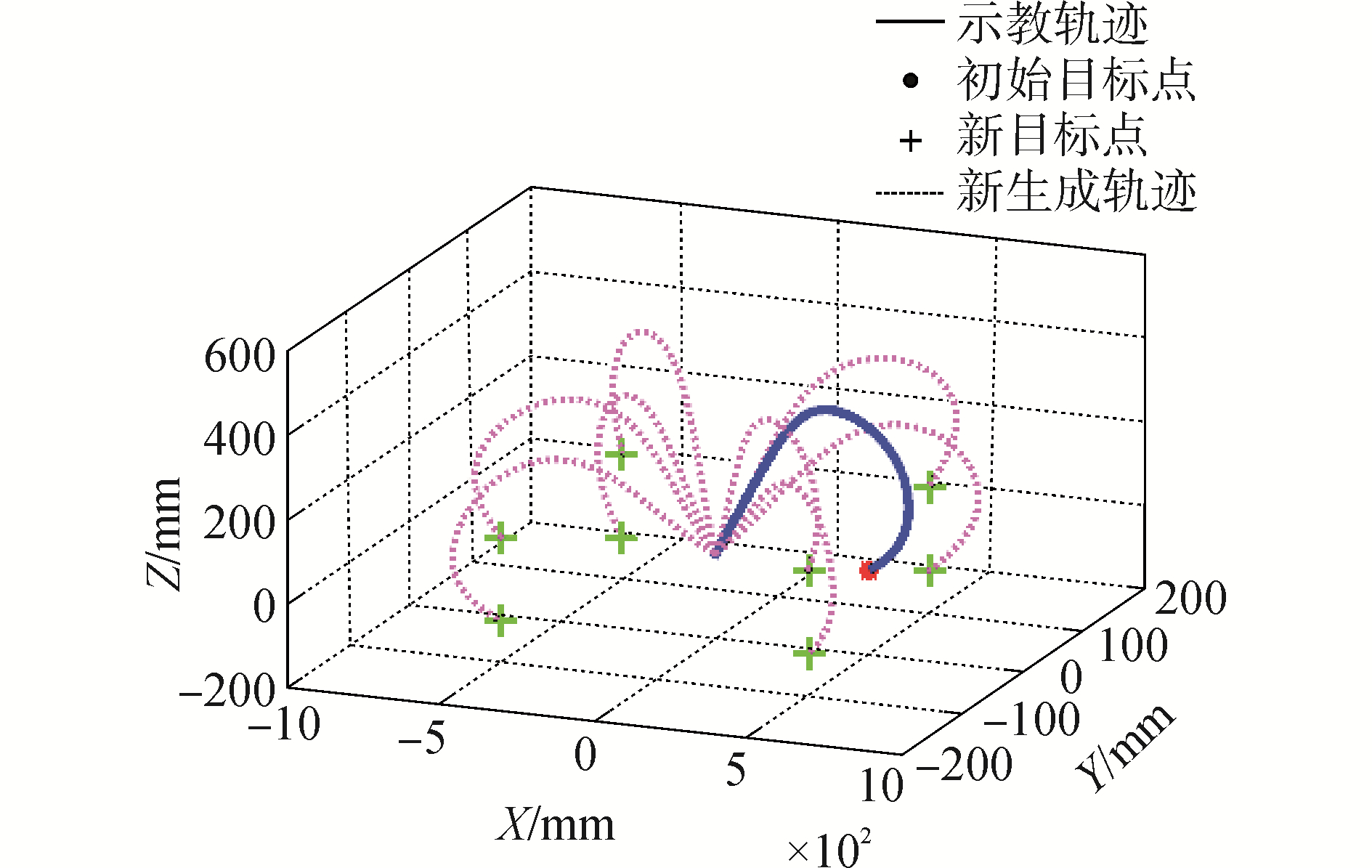Download: 图 1 基于DMP模型的轨迹生成方法 Fig. 1 Trajectory generation based on DMP model
2 基于距离加权k-NN算法的轨迹修正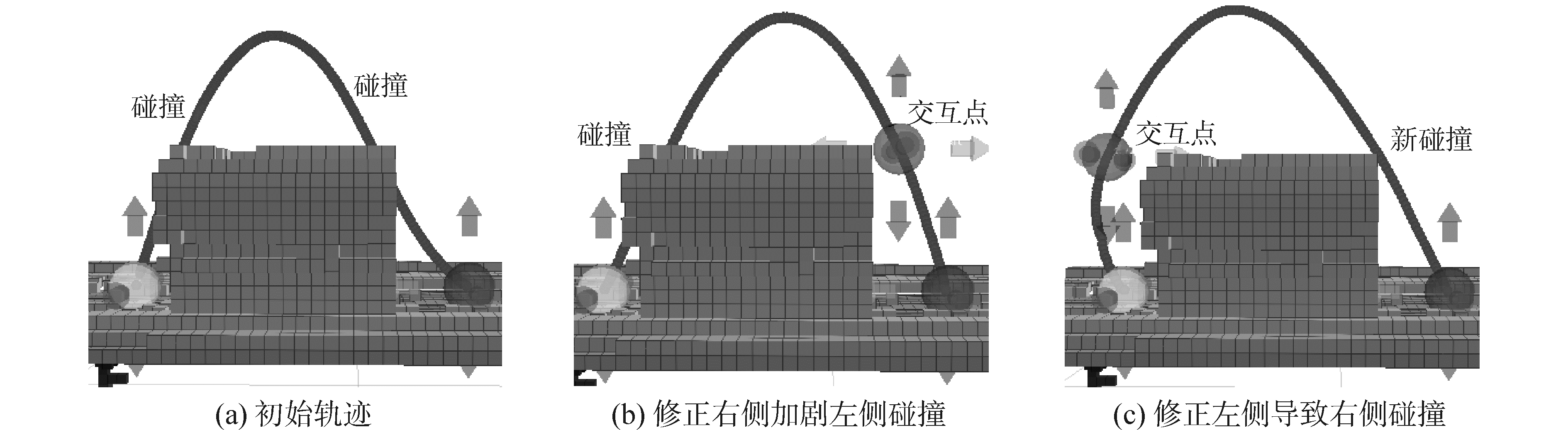Download: 图 2 基于双抛物线插值法的轨迹修正方法 Fig. 2 Trajectory adjustment based on dual parabolic interpolation

2.1 基于距离加权k-NN的轨迹修正算法

 $T = {T_{{\rm{original}}}} + \Delta T$ (4)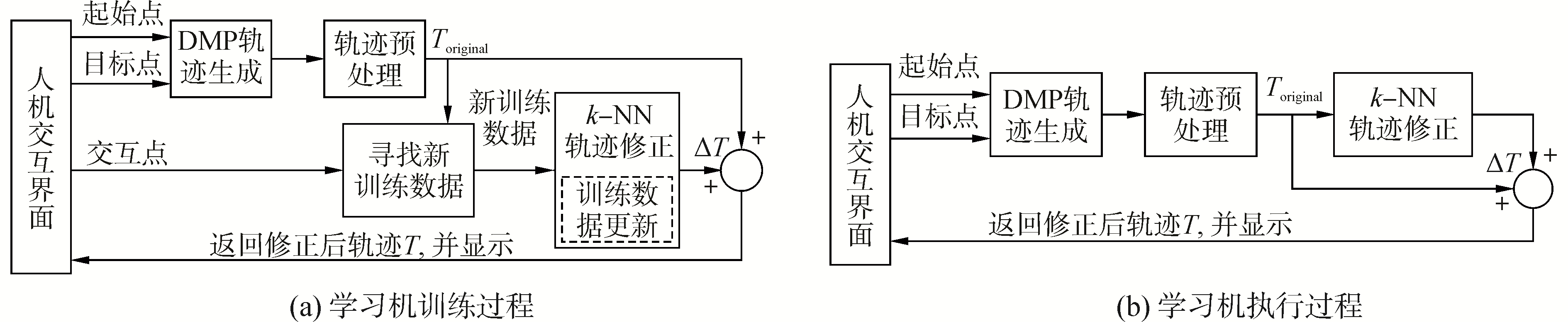Download: 图 3 轨迹修正过程 Fig. 3 Trajectory adjustment process

1) 计算轨迹点Pi到每一个训练数据Pk的距离。本文采用欧式距离‖Pi-Pk‖；

2) 按照距离由小到大对训练数据进行排序；

3) 确定最近邻域个数K，本文暂定为10；

4) 按照反距离加权法计算轨迹点Pi的修正值ΔPi。其中，每个训练数据的权值与到该轨迹点的距离成反比。

 ${\omega _k} = {{\rm{e}}^{ - \lambda \left\| {{P_i} - {P_k}} \right\|}}/\left( {\sum\limits_{k = 1}^K {{{\rm{e}}^{ - \lambda \left\| {{P_i} - {P_k}} \right\|}}} } \right)$ (5)

 $\Delta {P_i} = \sum\limits_{k = 1}^K {({\omega _k}{d_k})}$ (6)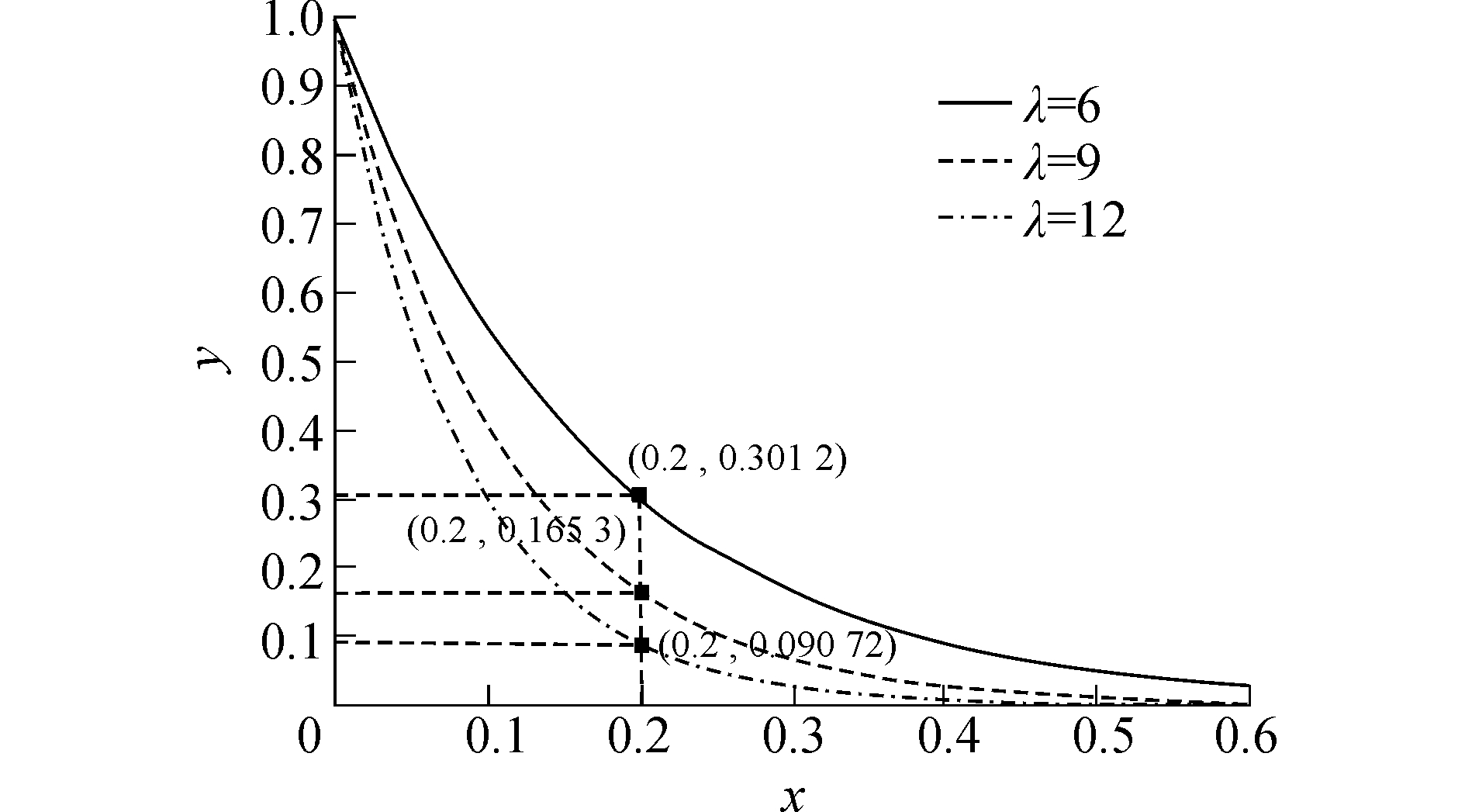Download: 图 4 y=exp(-λx)的函数关系曲线图 Fig. 4 The functional relationship graphic of y=exp(-λx)

 $\Delta {P_i} = \sum\limits_{k = 1}^K {\left( {\frac{1}{{1 + {{\rm{e}}^{\alpha (\left\| {{P_i} - {P_k}} \right\| - {P_{{\rm{threshold}}}})}}}}{\omega _k}{d_k}} \right)}$ (7)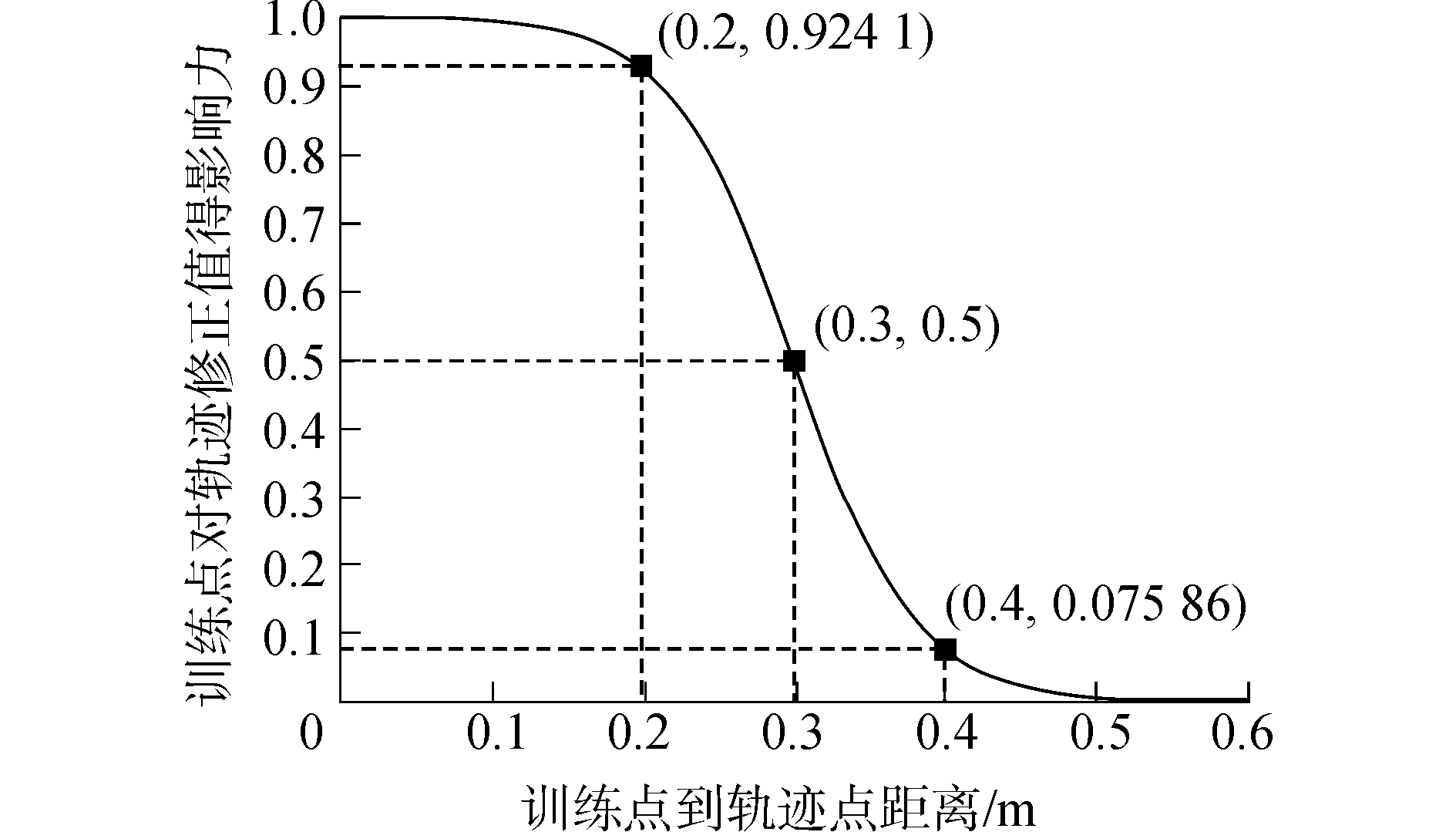Download: 图 5 训练点到轨迹点距离与该训练点对轨迹修正值影响力的关系 Fig. 5 The relationship between the distance from training data to the trajectory point and the influence of the training data on the adjustment

 $\begin{array}{*{20}{c}} {\Delta {P_i} = }\\ {\sum\limits_{k = 1}^K {\left( {\frac{1}{{1 + {{\rm{e}}^{\beta (\left\| {{R_i} - {r_k}} \right\| - {R_{{\rm{threshold}}}})}}}}\frac{1}{{1 + {{\rm{e}}^{\alpha (\left\| {{P_i} - {P_k}} \right\| - {P_{{\rm{threshold}}}})}}}}{\omega _k}{d_k}} \right)} } \end{array}$ (8)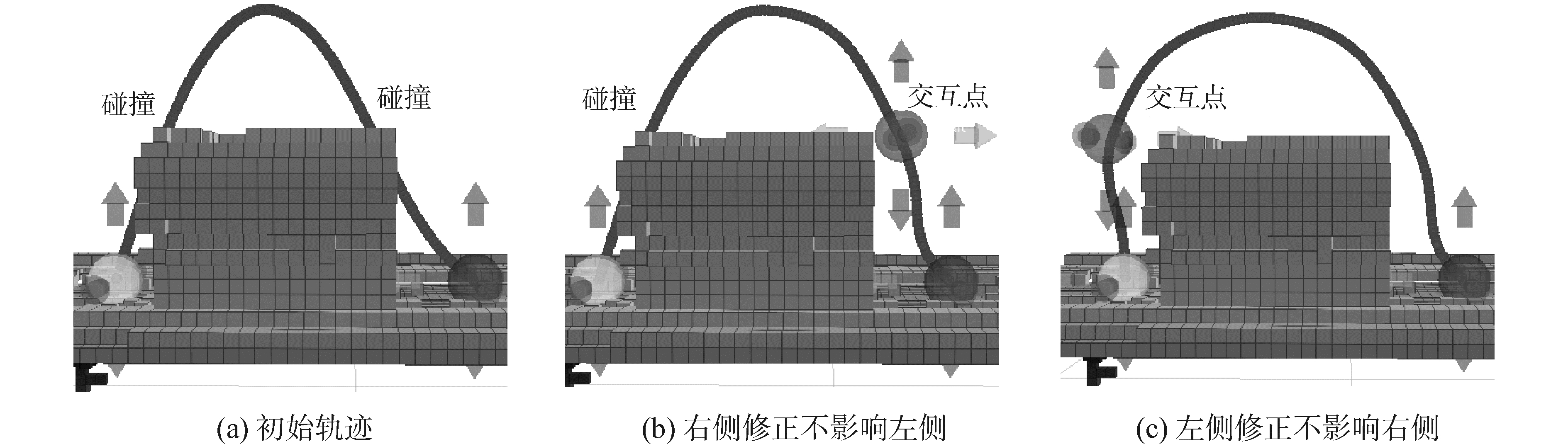Download: 图 6 基于k-NN的轨迹修正方法 Fig. 6 Trajectory adjustment based on k-NN

3 训练数据的更新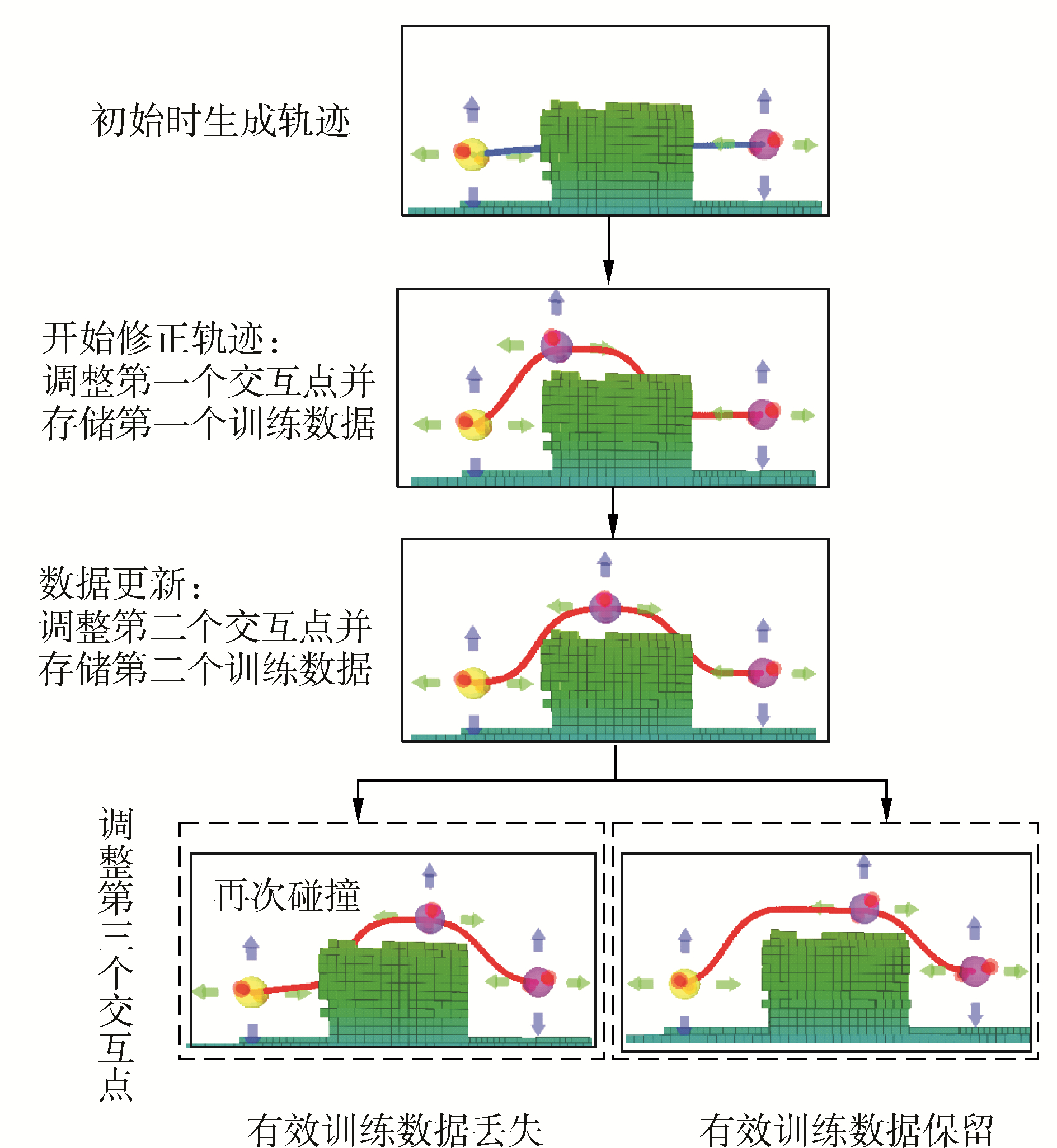Download: 图 7 训练数据的更新 Fig. 7 Training data updating and selection

FOR k=1：1：M

data_new(k)=data(M-k+1)；

ENDFOR

num=M；   \\需要具有两个或两个以上训练数据

FOR i=1:1：num-1

FOR j=i+1:1：num

IF (‖ pj- pi‖ < δupdate )& (‖rj- ri‖ < δupdate )

DELETE data_new(j) \\删除该训练数据

ENDIF

ENDFOR

ENDFOR

4 避障能力实验 4.1 实验平台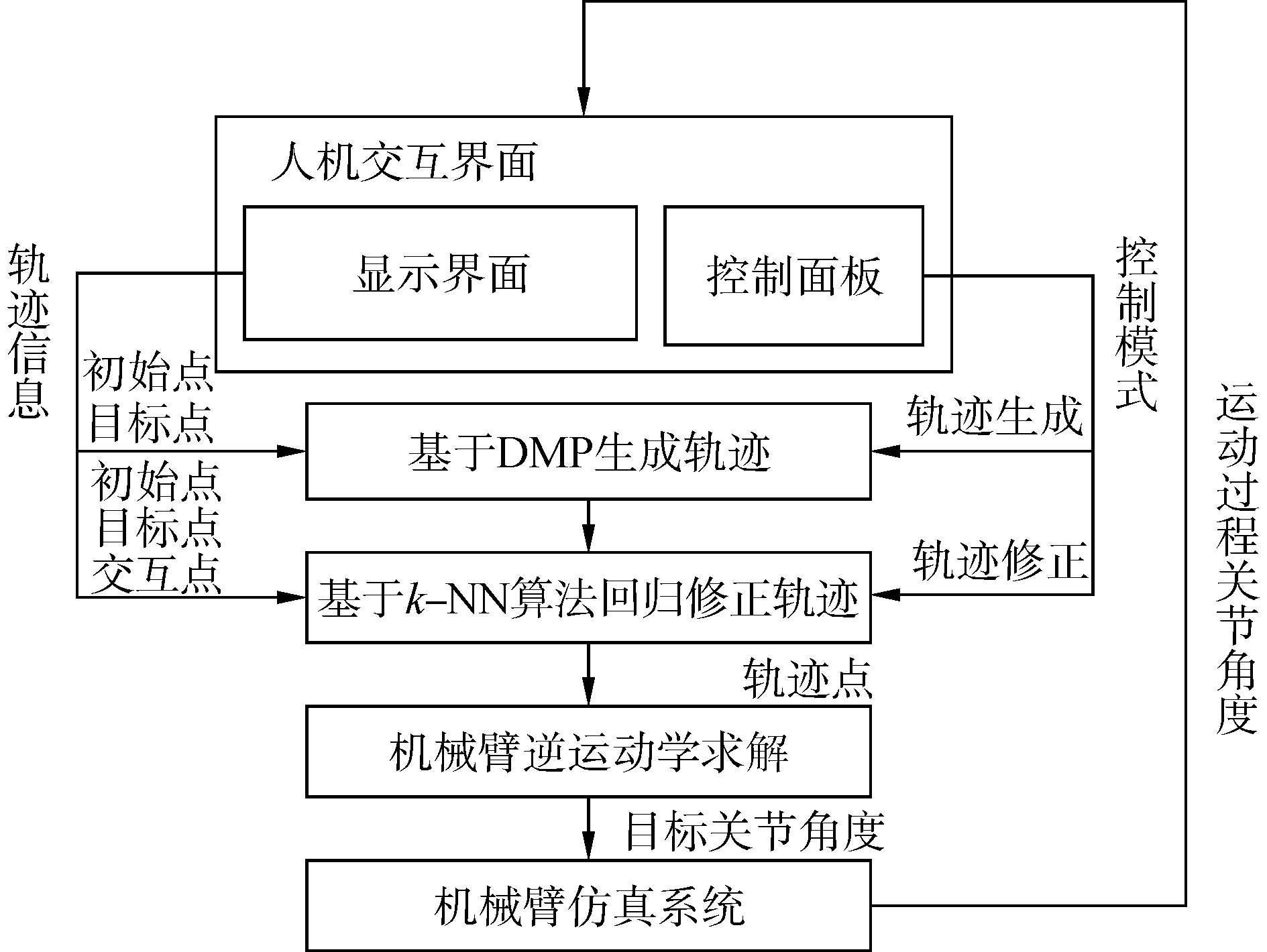Download: 图 8 模块间通信体制 Fig. 8 Communication Scheme between modules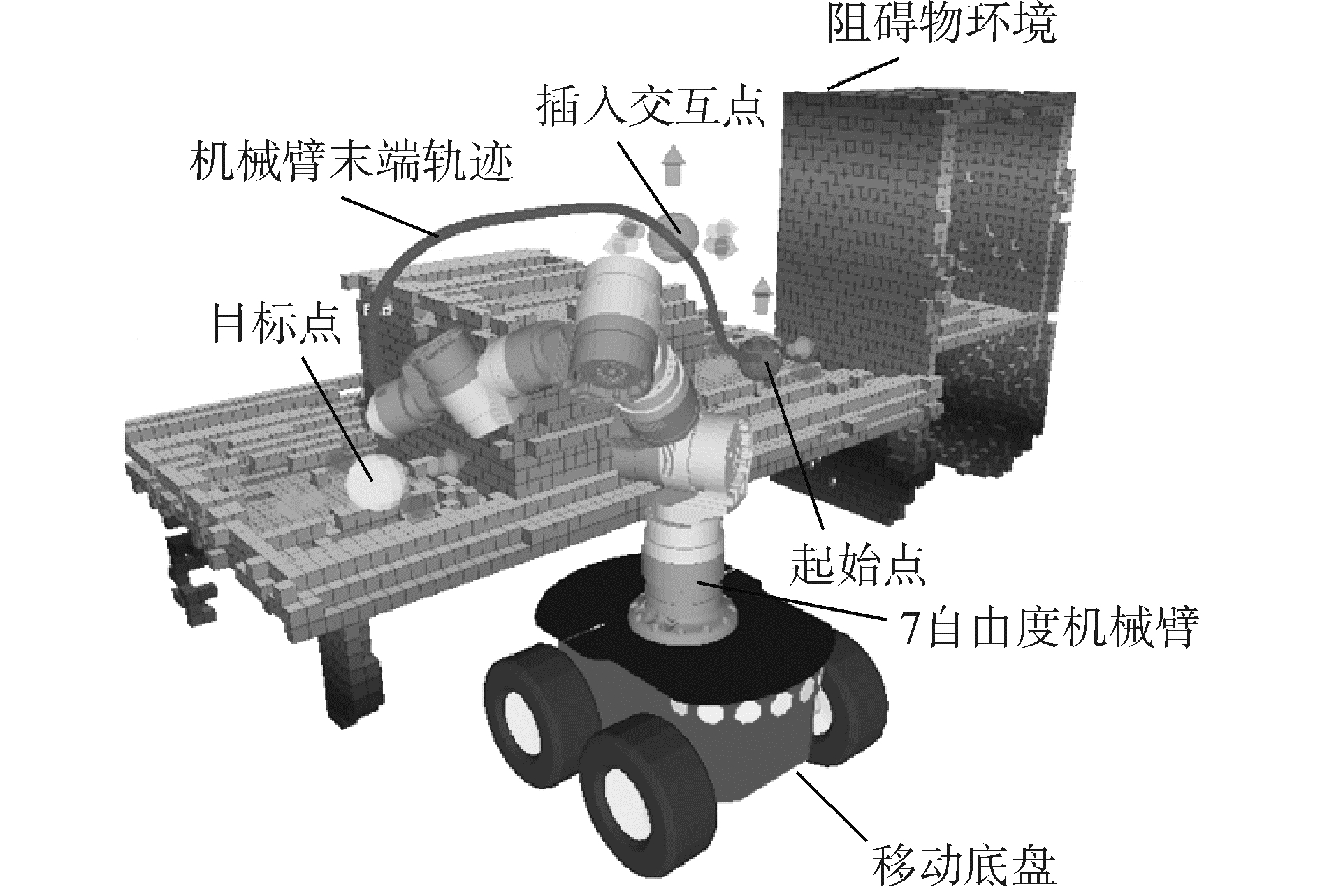Download: 图 9 人机交互界面 Fig. 9 Interface for human robot interaction
4.2 避障能力测试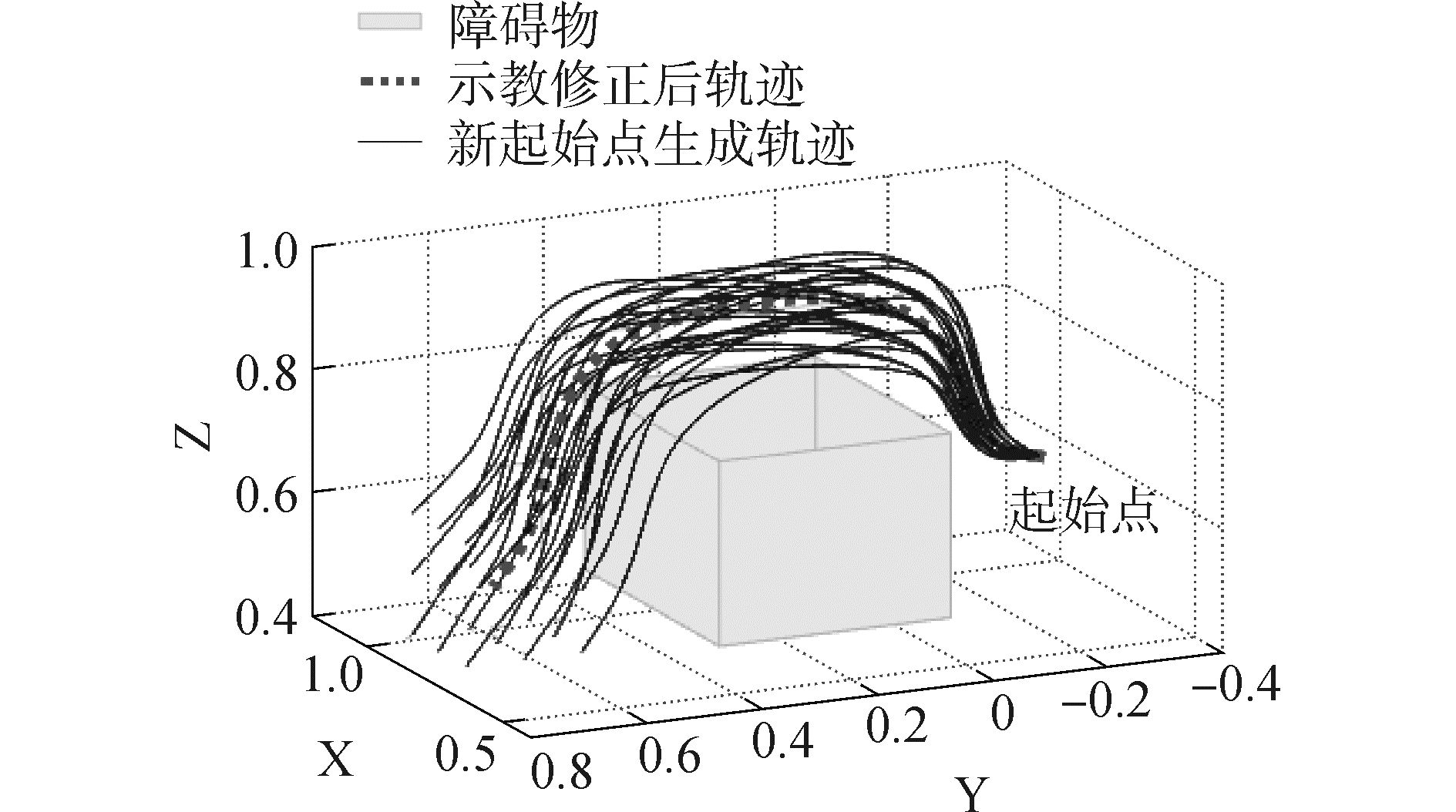Download: 图 10 机器人相对障碍物环境静止时，轨迹避障实验 Fig. 10 Obstacle avoidance experiment of the trajectory in the case that robot is relative static to the obstacle environment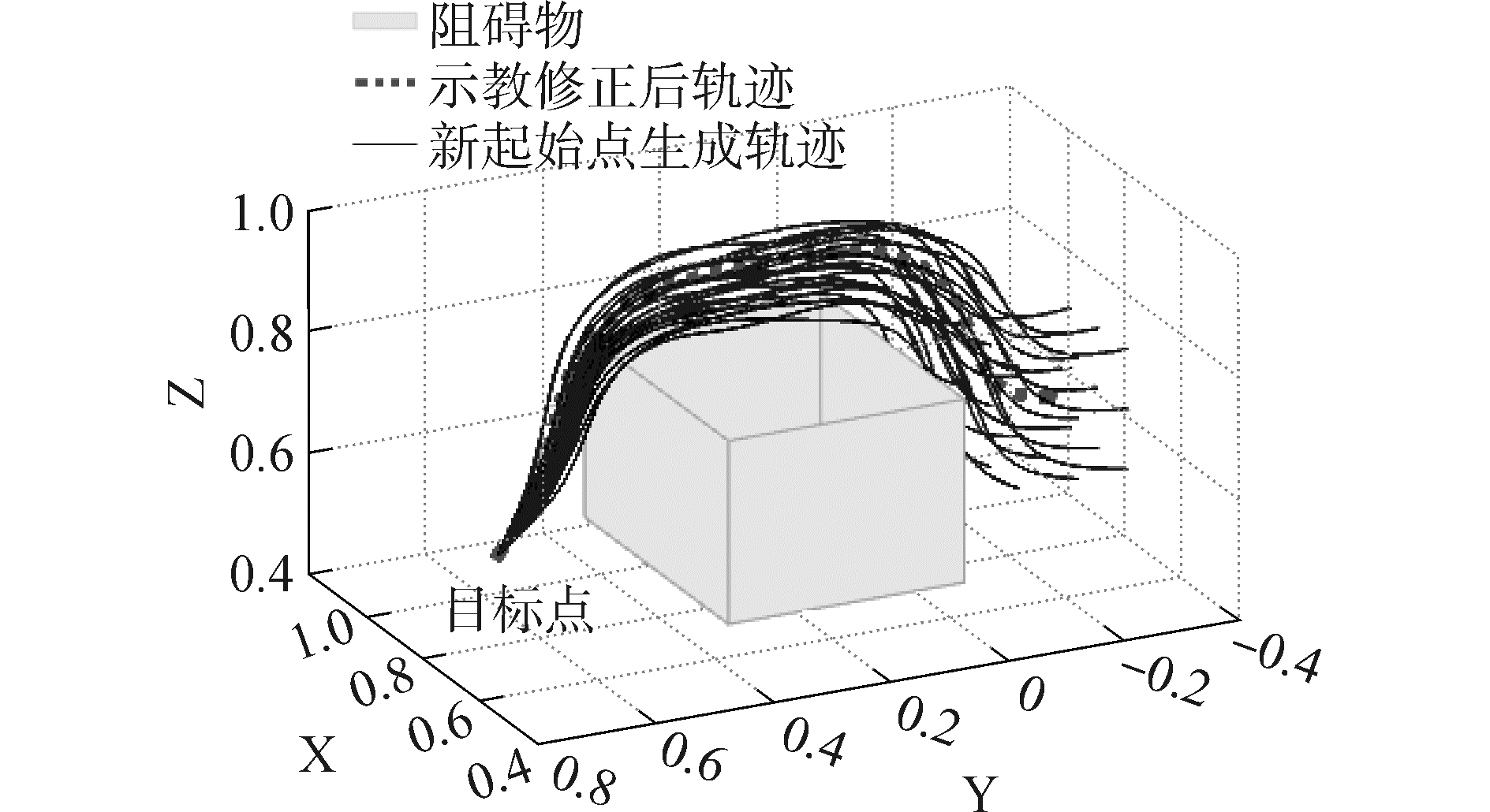Download: 图 11 机器人跟随初始点移动时，轨迹避障实验 Fig. 11 Obstacle avoidance experiment of the trajectory in the case that robot is following the position of start pointDownload: 图 12 避障决策实验 Fig. 12 Experiment of making obstacle avoidance decision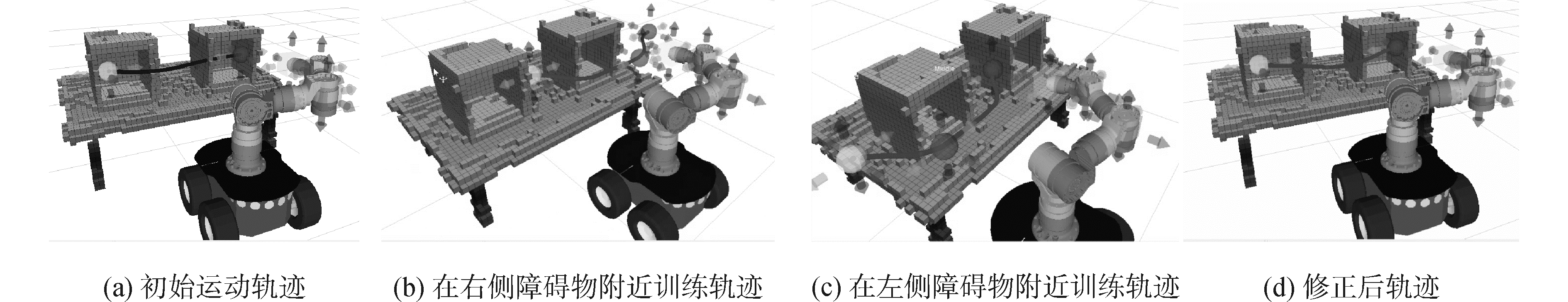Download: 图 13 多障碍物场景的信息融合 Fig. 13 Information fusion of multiple obstacle scenariosDownload: 图 14 机械臂沿轨迹运动 Fig. 14 Manipulator moves along the trajectory
4.3 避障算法的实时性测试表 1 实时性测试数据 Tab.1 Real-time performance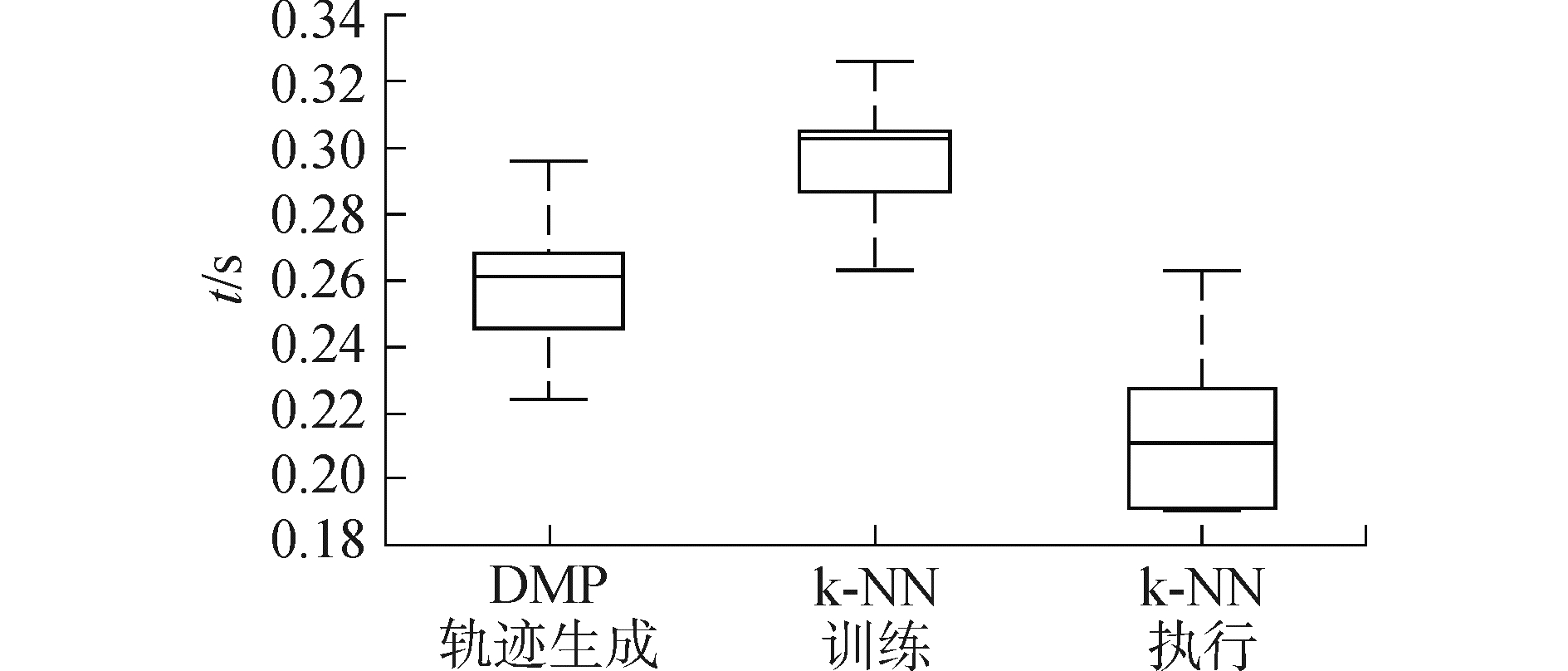Download: 图 15 实时性测试的箱形图 Fig. 15 Box-plot of the real-time performance

6 结论

1) 通过友好的人机交互界面，操作者可以利用其视觉感知和避障能力向机器人系统示教轨迹修正过程。不仅提高了系统的控制直观性，而且障碍物的环境信息和操作者轨迹修正策略的倾向性均隐性的融合于示教信息中，避免了对该障碍物环境的准确建模和信息提取。

2) 结合Sigmoid函数提出改进的距离加权k-近邻算法用于障碍物环境下的轨迹修正。解决与距离相关的训练点影响力问题和不同机器人位置下的避障决策问题。与全局的双抛物线插值算法相比，该算法不仅具有更好的灵活性，而且可通过学习将训练点的避障能力拓展到新工作空间，从而避免大量的重复性训练工作，减轻操作者的使用负担，并通过算法的实时性分析验证了该算法的在线修正能力。

3) 提出新的训练数据在线更新方法，一方面保证了系统的在线更新能力，另一方面避免了潜在有效训练点的丢失。从而提高训练数据的质量，保证隐含环境信息的准确性，使系统的应用灵活性和适应性得以提高。

  WREDE S, BEYER O, DREYER C, et al. Vertical integration and service orchestration for modular production systems using business process models[J]. Procedia technology, 2016, 26: 259-266. DOI:10.1016/j.protcy.2016.08.035 (0)  PARK D H, HOFFMANN H, PASTOR P, et al. Movement reproduction and obstacle avoidance with dynamic movement primitives and potential fields[C]//Humanoids 2008-8th IEEE-RAS International Conference on Humanoid Robots. Daejeon, South Korea, 2008: 91-98. http://ieeexplore.ieee.org/xpls/icp.jsp?arnumber=4755937 (0)  LOZANO-PEREZ T. A simple motion-planning algorithm for general robot manipulators[J]. IEEE journal on robotics and automation, 1987, 3(3): 224-238. DOI:10.1109/JRA.1987.1087095 (0)  LOPEZ A S, ZAPATA R, LAMA M A O. Sampling-based motion planning:a survey[J]. Computación Y sistemas, 2008, 12(1): 5-24. (0)  PAN J, SUCAN I A, CHITTA S, et al. Real-time collision detection and distance computation on point cloud sensor data[C]//2013 IEEE International Conference on Robotics and Automation. Karlsruhe, Germany, 2013: 3593-3599. http://ieeexplore.ieee.org/xpls/icp.jsp?arnumber=6631081 (0)  WREDE S, EMMERICH C, GRVNBERG R, et al. A user study on kinesthetic teaching of redundant robots in task and configuration space[J]. Journal of human-robot interaction, 2013, 2(1): 56-81. DOI:10.5898/JHRI.1.2 (0)  CALINON S, GUENTER F, BILLARD A. On learning, representing, and generalizing a task in a humanoid robot[J]. IEEE transactions on systems, man, and cybernetics, part B (cybernetics), 2007, 37(2): 286-298. DOI:10.1109/TSMCB.2006.886952 (0)  BILLARD A, GROLLMAN D H. Robot learning by demonstration[J]. Scholarpedia, 2013, 8(12): 3824. DOI:10.4249/scholarpedia.3824 (0)  STEIL J J, EMMERICH C, SWADZBA A, et al. Kinesthetic teaching using assisted gravity compensation for model-free trajectory generation in confined spaces[M]//RÖHRBEIN F, VEIGA G, NATALE C. Gearing Up and Accelerating Cross-Fertilization Between Academic and Industrial Robotics Research in Europe. Cham: Springer, 2014: 107-127. (0)  FISCHER K, KIRSTEIN F, JENSEN L C, et al. A comparison of types of robot control for programming by Demonstration[C]//2016 11th ACM/IEEE International Conference on Human-Robot Interaction. Karlsruhe, Germany, 2016: 213-220. http://dl.acm.org/citation.cfm?id=2906868 (0)  刘维惠, 陈殿生, 张立志. 人机协作下的机械臂轨迹生成与修正方法[J]. 机器人, 2016, 38(4): 504-512. LIU Weihui, CHEN Diansheng, ZHANG Lizhi. Trajectory generation and adjustment method for robot manipulators in human-robot collaboration[J]. Robot, 2016, 38(4): 504-512. (0)  UDE A, GAMS A, ASFOUR T, et al. Task-specific generalization of discrete and periodic dynamic movement primitives[J]. IEEE transactions on robotics, 2010, 26(5): 800-815. DOI:10.1109/TRO.2010.2065430 (0)  KULVICIUS T, NING K J, TAMOSIUNAITE M, et al. Joining movement sequences:modified dynamic movement primitives for robotics applications exemplified on handwriting[J]. IEEE transactions on robotics, 2012, 28(1): 145-157. DOI:10.1109/TRO.2011.2163863 (0)  RAI A, MEIER F, IJSPEERT A, et al. Learning coupling terms for obstacle avoidance[C]//2014 14th IEEE-Ras International Conference on Humanoid Robots. Madrid, Spain: IEEE, 2014: 512-518. http://ieeexplore.ieee.org/xpls/abs_all.jsp?arnumber=7041410 (0)  RAI A, SUTANTO G, SCHAAL S, et al. Learning feedback terms for reactive planning and control[C]//2017 IEEE International Conference on Robotics and Automation. Singapore, Singapore, 2017: 2184-2191. http://ieeexplore.ieee.org/document/7989252/ (0)  KPOTUFE S. k-NN regression adapts to local intrinsic dimension[C]//Advances in Neural Information Processing system.Granda, spain, 2011: 729-737. http://cn.arxiv.org/abs/1110.4300 (0)  DUDANI S A. The distance-weighted k-Nearest-Neighbor rule[J]. IEEE transactions on systems, man, and cybernetics, 1976, SMC-6(4): 325-327. DOI:10.1109/TSMC.1976.5408784 (0)  CHA E, KRONANDER K, BILLARD A. Combined kinesthetic and simulated interface for teaching robot motion models[C]//2015 24th IEEE International Symposium on Robot and Human Interactive Communication. Kobe, Japan, 2015: 83-88. http://ieeexplore.ieee.org/xpls/abs_all.jsp?arnumber=7333655 (0)  FANG B, GUO D, SUN F C, et al. A robotic hand-arm teleoperation system using human arm/hand with a novel data glove[C]//2015 IEEE International Conference on Robotics and Biomimetics. Zhuhai, China, 2015: 2483-2488. (0)